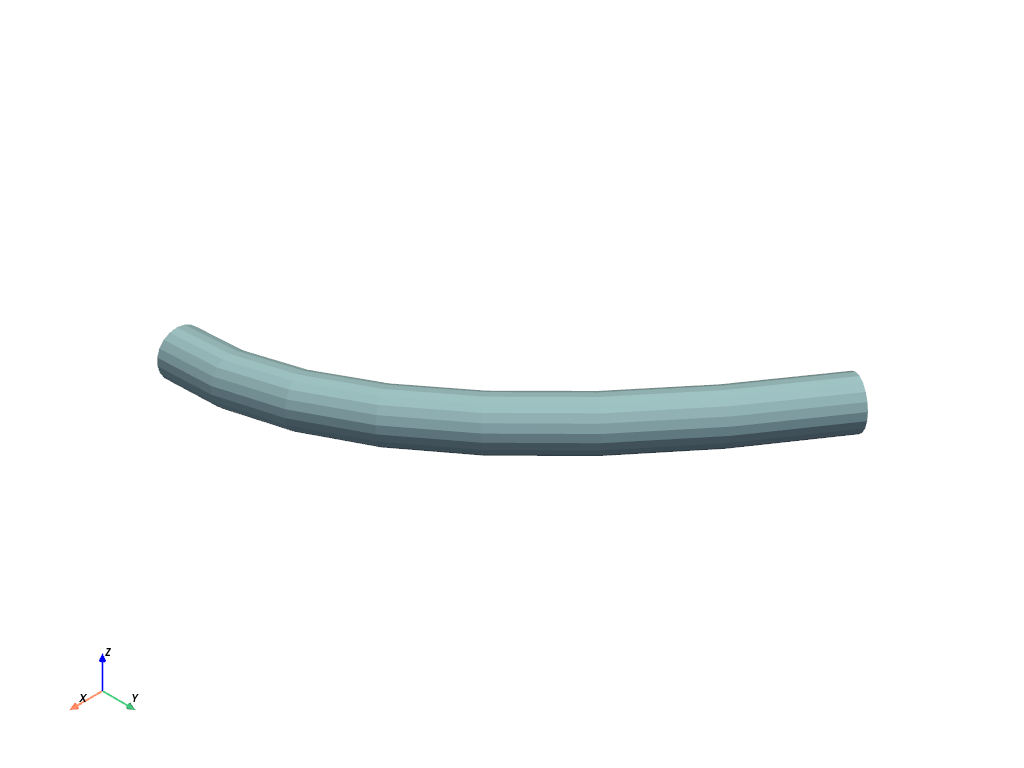# Points to Tube¶

This example will demonstrate how to to build a tube from a set of points in vtkPolyData.

Takes points from a vtkPolyData object and constructs a line of those points then builds a polygonal tube around that line with some specified radius and number of sides.

```# sphinx_gallery_thumbnail_number = 2
import pyvista
import numpy as np
from PVGeo.filters import PointsToTube
```
```def path(y):
"""Equation: x = a(y-h)^2 + k"""
a = - 110.0 / 160.0**2
x = a*y**2 + 110.0
return x, y

x, y = path(np.arange(0.0, 200.0, 25.0))
zo = np.linspace(9.0, 11.0, num=len(y))
points = pyvista.PolyData(np.c_[x,y,zo])

points.plot(point_size=10)
```Out:

```[(288.4271366070708, 331.7230350445708, 254.2230350445708),
(44.2041015625, 87.5, 10.0),
(0.0, 0.0, 1.0)]
```
```# Use the filter: here is vtkPolyData containing the tube
tube = PointsToTube(nearestNbr=True).apply(points)
tube.plot(color=True)
```Out:

```[(310.504116595436, 355.2706516584843, 274.2397677001663),
(46.26434889525197, 91.03088395830022, 9.999999999982224),
(0.0, 0.0, 1.0)]
```

Total running time of the script: ( 0 minutes 0.499 seconds)

Gallery generated by Sphinx-Gallery